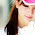## Random walks and random stumbles

Random walks are a gold mine for a wide variety of stochastic theory and practice. They are easy to explain, easy to code, and easy to misunderstand. In this section, we start out with the simplest imaginable random walk and then show how things can go wrong. Note that this post is also available in nbviewer where the math below may render better than here in blogger.

Let's examine the problem of the one-dimensional random walk. Consider a particle at the origin ($x=0$), with $p$ probability of moving to the right and $q=1-p$ probability of moving to the left. When the particle reaches $x=1$, the experiment terminates. On average, how many steps are required for this to terminate if $p=1/2$? This seems like a reasonable question, doesn't it?

The first thing we have to do is establish the conditions under which termination occurs. Thus, we need the probability that the particle ultimately reaches $x=1$. We'll call this probability $P$. On average, how many steps does it make to terminate?

This experiment is easy enough to code as shown below:
In :
def walk():
'starting at x=0, step left/right with probability 1/2 until x=1'
x=0
while x!=1:
x+=random.choice([-1,1]) # equi-probable left-right move
yield x

Some classical deft reasoning leads to:
$$P = p + q P^2$$
where $p$ is the probability it moves to $x=1$ from $x=0$ on the first try, and $q$ is the probability it does not, but then ultimately makes it back to $x=0$ (with probability $P$) and then again makes it from there to $x=1$, again with probability $P$ (thus, $P^2$).
There are two solutions to $P$, $P=1$ and $P=p/(1-p)$. The crossover point is $p=1/2$. This is drawn below.
In :
from __future__ import  division # want floating point division

fig,ax=subplots()
p = linspace(0,0.5,20)
ax.plot(p,p/(1-p),label=r'$p<1/2$',lw=3.)
ax.plot([0.5,1],[1,1],label=r'$p > 1/2$',lw=3.)
ax.axis(ymax=1.1)
ax.legend(loc=0)
ax.set_xlabel('$p$',fontsize=16)
ax.set_ylabel('$P$',fontsize=16)
ax.set_title(r'Probability of reaching $x=1$ from $x=0$',fontsize=16)
ax.grid()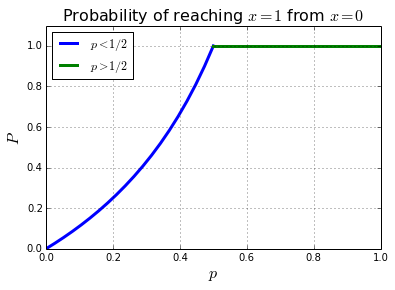## Average number of steps to termination

A straight-forward question to ask is what is the average number of steps it takes to ultimately terminate this random walk? The quick analysis above says that the particle will ultimately terminate, but, on average, how many steps are required for this to happen?

Unfortunately, the analysis above is not very helpful here because the statement is about the probability of ultimate termination, not the probability of termination given a particular point somewhere on the left of the origin.

Fortunately, we have all the Python-based tools to experimentally get at this. The following generator describes the $p=1/2$ random walker.

In :
def walk():
'starting at x=0, step left/right with probability 1/2 until x=1'
x=0
while x!=1:
x+=random.choice([-1,1]) # equi-probable left-right move
yield x

Let's try generating a realization of this walk.
In :
random.seed(123) #  set seed for reproducibility

s = list(walk()) # generate the random steps

fig,ax=subplots()
ax.plot(s)
ax.set_ylabel("particle's x-position",fontsize=16)
ax.set_xlabel('step index k',fontsize=16)
ax.set_title('Example of random walk',fontsize=16)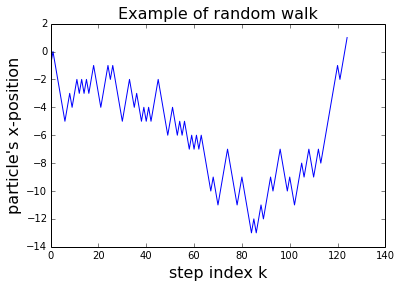Now, that we're set up, we can generate a whole list of these walks and then average their lengths to estimate the mean of these walks.

In :
s = [list(walk()) for i in range(50)] # generate 50 random walks
len_walk=map(len,s) # lengths of each walk

The following plots out a few of these random walks so we can get a feel of what's going on with the average length.

In :
fig,ax=subplots()
for i in s:
ax.plot(i)
ax.set_ylabel("particle's x-position",fontsize=16)
ax.set_xlabel('step index k',fontsize=16)
ax.set_title('average length=%3.2f'%(mean(len_walk)))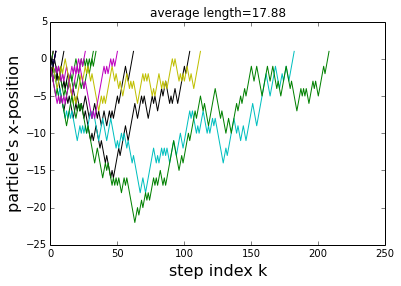Now, here's where things get interesting! Fifty samples of the random walk is really not that many, so we'd like to hopefully get a better average by generating a lot more. If you try to generate, say, 1000 realizations, you'd be in for a long wait! This is because some of the random walks are really, really long! Furthermore, this is a persistent phenomenon; it's not just a bad draw from the random deck. Even if there is only one really long walk, it seriously distorts the average. It's tempting to conclude that this is just some outlier and get on with it, but not doing so will lead us to a very powerful theorem in stochastic processes.

To get a better picture of what's going on here, let's re-define our random walker function so we can limit how far it can go and thereby how long we'd have to wait.

In :
def walk(limit=50):
'limited version of random walker'
x=0
while x!=1 and abs(x)<limit:
x+=random.choice([-1,1])
yield x


Because we really just want to count the walks, we can save memory by not collecting the individual steps and just reporting the length of the walk.

In :
def nwalk(limit=500):
'limited version of random walker. Only returns length of path, not path itself'
n=x=0
while x!=1 and n<limit:
x+=random.choice([-1,1])
n+=1
return n # return length of walk

In :
len_walk = [nwalk() for i in range(500)] # generate 500 limited random walks


The usual practice would be to draw a histogram (i.e. an approximation of the probability density) here, but sometimes the automatic binning makes things hard to see. Instead, a cumulative distribution plot is helpful here. The excellent pandas module and some very useful data structures for this kind of work.

In :
import pandas as pd
from collections import Counter, OrderedDict

lw = pd.Series(Counter(len_walk))/len(len_walk)
fig,ax=subplots()

ax.plot(lw.index,lw.cumsum(),'-o',alpha=0.3)
ax.axis(xmin=-10,ymin=0)
ax.set_title('Estimated Cumulative Distribution',fontsize=16)
ax.grid()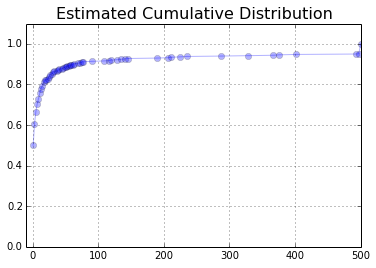What's interesting about the above plot is how steep the slope is. For a path length of one (terminating at the first step), we already have 50% of the probability accounted for. By a path-length of 100, we already have about 90% of the probability. The problem is squeezing out the remaining probability mass means computing random walks longer than 500 (our arbitrary limit). We can do all the above steps for higher limits far above 500, but this observation still holds. Thus, the problem with averaging this is that getting more probability further out competes with the lengths of those further paths. For the average to converge, we want to asymptotically get more improbable paths relatively faster than those paths grow!

Let's examine the standard deviation of our averages.
In :
def estimate_std(limit=10,ncount=50):
'quick estimate of the standard deviation of the averages'
ws= array([[ nwalk(limit) for i in range(ncount)] for k in range(ncount)])
return (limit,ws.mean(), ws.mean(1).std())

for limit in [10,20,50,100,200,300,500,1000]:
print 'limit=%d,\t average = %3.2f,\t std=%3.2f'% estimate_std(limit)

limit=10,  average = 4.15,  std=0.55
limit=20,  average = 6.25,  std=1.08
limit=50,  average = 10.00,  std=2.36
limit=100,  average = 15.55,  std=3.52
limit=200,  average = 21.59,  std=7.20
limit=300,  average = 25.60,  std=8.70
limit=500,  average = 32.20,  std=12.71
limit=1000,  average = 47.38,  std=21.40


If this were converging, then the standard deviation of the mean (and the mean itself) should start converging as the walk limit increased. This is obviously not happening here.

## Graph-based combinatorial approach

So far, we have been looking at this using samples and started suspecting that there is something going on with the convergence of the average. However, this is not uncovering what's going on under the sheets with the convergence of the average. Yes, we've tinkered with varying the sample size, but it could still be the case that there is some much larger sample size out there that would cure all the problems we have so far experienced.

The next code-block assembles some drawing utilities on the excellent networkx package so we can analyze this problem using graph combinatoric algorithms.

In :
import networkx as nx
import itertools as it

class Graph(nx.DiGraph):
'''
operations assuming pos attribute in nodes to support drawing and
manipulating path lattice.
'''
def draw(self, ax=None,**kwds):
'''
Draw based on pos attribute and pass kwds to nx.draw
:param: axes(optional, default is None)
'''
pos = self.get_pos()
node_size=kwds.pop('node_size',200)
alpha=kwds.pop('alpha',0.3)
if ax is None: nx.draw(self,pos=pos,
node_size=node_size,
alpha=alpha,
**kwds)
else:          nx.draw(self,pos=pos,
node_size=node_size,
alpha=alpha,
ax=ax,**kwds)

def get_pos(self,n=None):
'''
n := str name of node
get positions as returned dictionary
'''
pos=dict([( i,j['pos'] ) for i,j in self.nodes(data=True)])
if n is None:
return pos
else:
return pos[n]

def getx(self,x):
return sorted([(i,i['val'] ) for i in self.nodes(True) if i==x])

def gety(self,y):
return sorted([(i,i['val'] ) for i in self.nodes(True) if i==y])

# functions to allow diagonal lattice walking
def diagwalk(level,n):
x = level
y = -level
while y<=1 and x<n+1:
yield (x,y)
x+=1
y+=1

def diagwalker(n):
'daisy-chains the individual diagonal walkers'
assert n%2 # odd only
return it.chain(*(diagwalk(i,n) for i in range(n+1)))



This next code-block constructs the lattice graph that we'll explain below.

In :
def construct_graph(level=5):
g=Graph()
g.level = level

for x,y in g.nodes():
if y!=1 and x< g.level:

g.node[(0,0)]['val']=1L # long int
for j in diagwalker(g.level):
if j==(0,0): continue
x,y=j
g.node[j]['val']=sum(g.node[k]['val'] for k in g.predecessors(j))
return g
g=construct_graph()



The figure below shows a directed graph with the individual pathways the particle can take from $(0,0)$ to a particular end. The notation $(k,j)$ means that at iteration $k$, the particle is at $x=-j$ on the line. For example, all paths start at $(0,0)$ and there is only one path from $(0,0)$ to $(1,1)$. Likewise, the next terminus is at $(3,1)$, which is the pathway corresponding to reaching $x=1$ in three steps. There is only one path on the digraph that leads here. However, in this case, there are many pathways that are three-steps long, but that do not reach the terminus. For example, $(3,-1)$ is a path that leads to $x=-1$ in three steps.

Let's use some of the powerful algorithms in networkx to pursue these ideas.

In :
fig,ax=subplots()
fig.set_size_inches((6,6))
ax.set_aspect(1)
ax.set_title('Directed Path Lattice',fontsize=18)
g.draw(ax=ax)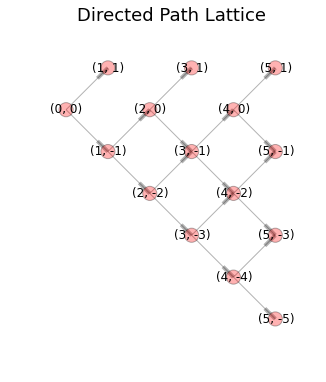The lattice diagram above shows the potential paths to termination for the random walk. For example, the all paths that lead to the node labeled (5,1) are those paths that take exactly five steps to terminate. Note that this is a directed graph so the graph can only be tranversed in the direction of the arrows (arrowheads denoted by thick ends as shown). Fortunately, networkx has powerful tools for computing these paths as shown in the following.

In :
l5=list(nx.all_simple_paths(g,(0,0),(5,1)))
for i in l5:
print i

fig,ax=subplots()
fig.set_size_inches((6,6))
g.draw(ax,with_labels=0)
g.subgraph(l5).draw(ax=ax,with_labels=1,node_color='b',node_size=700)
g.subgraph(l5).draw(ax=ax,with_labels=1,node_color='b',node_size=800)
ax.set_title('Pathways that terminate in five steps',fontsize=16)

[(0, 0), (1, -1), (2, 0), (3, -1), (4, 0), (5, 1)]
[(0, 0), (1, -1), (2, -2), (3, -1), (4, 0), (5, 1)]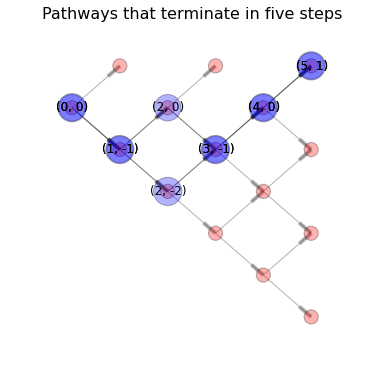The figure shows the two pathways that terminate in five steps. The other fact we need is how many pathways do not terminate in five steps, then we'll be on our way to computing a probability. Fortunately, we already have this built into our initial graph construction.

In :
for i in g.getx(5):
print 'node (%d,%d)\t'%(i),
print 'number of paths = %d'%(i)

node (5,-5) number of paths = 1
node (5,-3) number of paths = 4
node (5,-1) number of paths = 5
node (5,1) number of paths = 2


Now, we can compute the conditional probability of terminating in five steps as simply:
$$\mathbb{P}(5|\hat{3},\hat{1}) = \frac{\text{no. paths to (5,1)}}{\text{total no. of paths of length 5}}$$
This is a conditional probability because the only way to terminate in five steps is to not have terminated at step 1 or 3, which is emphasized by the hat on top of those digits in the above equation. To compute the unconditional probability, we need to account for
$$\mathbb{P}(\hat{3},\hat{1}) = \mathbb{P}(\hat{3}|\hat{1}) \mathbb{P}(\hat{1})$$
by computing this recursively,
$$\mathbb{P}(\hat{3}|\hat{1}) = 1- \mathbb{P}({3}|\hat{1})$$
where $\mathbb{P}(\hat{1})=1/2$. Plugging and chugging with the above result gives
$$\mathbb{P}(5) = \frac{1}{16}$$
Because this is tedious to do by hand, we can automate this entire process as shown below.
In :
import operator as op

def get_cond_prob(g):
'compute conditional probability for later'
cp = OrderedDict()
for i,j in g.gety(1):
if (i,-i ) not in g: continue
cp[i] = g.node[i]['val']/sum(map(op.itemgetter(1),g.getx(i)))
return cp

def get_prob(g,cp=None):
'get unconditional probability of termination '
prob = OrderedDict()
cq = OrderedDict()
if cp is None: cp = get_cond_prob(g)
for i,j in cp.iteritems(): cq[i]=1-j
prob=  0.5  # initial condition
for i in cp.keys():
prob[i]= cp[i]*prod(cq.values()[:(i-1)//2][::-1])
return prob


The following quick check matches the result we computed earlier for termination in exactly five steps.
In :
g=construct_graph(15)
p=get_prob(g)
print 'prob of termination in 5 steps = ',p

prob of termination in 5 steps =  0.0625


The following plot shows the probability of termination for each number of steps.
In :
fig,ax=subplots()
ax.axis(ymax=1)
ax.plot(p.keys(),p.values(),'-o')
ax.set_xlabel('exact no. of steps to terminate',fontsize=18)
ax.set_ylabel('probability',fontsize=18)
ax.axis(ymax=.6)

Out:
(0.0, 16.0, 0.0, 0.6)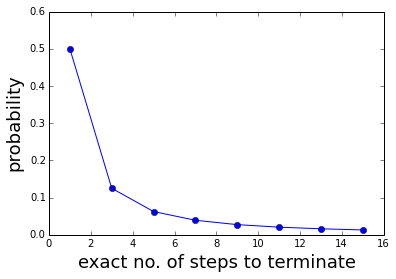Thus, we have a computational method for computing the probability of terminating in a given number of steps. What we need now is a separate analytical result that confirms our work so far. This is where the book by Feller comes in (Feller, William. An Introduction to Probability Theory and Its Applications: Volume One. John Wiley & Sons, 1950.).

## Using Generating Functions (Feller, Vol I, p. 271)

In random walk terminology, the probability that first visit to $x=1$ takes place at the nth step is denoted as $\phi_n$. Furthermore, the generating function is defined as:
$$\Phi(s) = \sum_{n=0}^\infty \phi_n s^n$$
we will need two other random variables. $N$ is the first time that the particle reaches $x=1$. This is a random variable with probability $\phi_n$. Likewise, $N_1$ is the number of trials required to move the particle from anywhere to the left of $x=-1$ to $x=0$. Also, $N_2$ is the number of trials required to move the particle from $x=0$ to $x=1$. Now, here comes the key step: these three random variables are independent with the same probability distribution. With these definitions, we have
$$N = 1 + N_1 + N_2$$
In other words, you need $N_1$ trials to make it from wherever the particle was on the left of the origin back to the origin; and then you need $N_2$ trials to make it from there to $x=1$. The first $1$ accounts for the first step to the left from the origin. Thus we have,
$$\mathbb{E}(s^N|X_1=-1) = \mathbb{E}(s^{1 + N_1 + N_2}|X_1=-1) = s\Phi(s)^2$$
where we can multiply through because of the mutual independence of the random variables. Additionally,
$$\mathbb{E}(s^N|X_1=1) = s$$
because $N_1 = N_2 = 0$ in this case. Unwinding the conditional expectation yields,
$$\mathbb{E}(s^N) = \mathbb{E}(s^N|X_1=1) p + \mathbb{E}(s^N|X_1=-1) q$$
Writing this out yields,
$$\Phi(s) = p s + s \Phi(s)^2 (1-p)$$
This is a quadratic equation in $\Phi(s)$, so we have two solutions:
$$\Phi_1(s) = \frac{-1-\sqrt{1+4 (p-1) p s^2}}{2 s(p -1 )}$$
and
$$\Phi_2(s) = \frac{-1+\sqrt{1+4 (p-1) p s^2}}{2 s(p -1 )}$$
How do we pick between them? The limit of $\Phi_2(s)$ is unbounded as $s \rightarrow 0$. Thus, $\Phi(s=1) = \Phi_1(s=1)$.
One of the properties of the $\Phi$ function is that $\Phi(s=1)$ should sum to one. In this case, we have
$$\Phi(s=1) = \Phi_1(s=1)= \frac{1-|p-q|}{2 q}$$
Thus, if $p < q$, we have
$$\sum \phi_n = p/q$$
which doesn't equal one. The problem here is that the definition of the random variable $N$ only considered the conclusion that the particle would ultimately reach $x=1$. The other possibility, which accounts for the missing probability here, is that the particle never terminates. Similarly, when $p \ge q$, we have
$$\sum \phi_n = 1$$
This should be no surprise because we saw this exact same result when we first started investigating this! Namely, $p \ge q \implies p \ge 1/2$, which is what we concluded from our first plot. This is the situation where the particle always terminates.
The first derivative of $\Phi(s)$ function evaluated at $s=1$ is the mean. Thus,
$$\bar{N} = \mathbb{E}(N) = \Phi'(s=1)= -\left( \frac{-1 + 4\,p\,q + {\sqrt{1 - 4\,p\,q}}} {-2\,q + 8\,p\,q^2} \right)$$
Note that this is unbounded when $p=1/2$ which is exactly what we have been observing experimentally.
To get at the individual probabilities for $p=1/2$, we can use a power series expansion on
$$\Phi(s)\bigg|_{p=1/2}=-\left( \frac{-1 + {\sqrt{1 - s^2}}}{s} \right)$$
Using the binomial expansion theorem, this gives
$$\phi_{2k-1} = (-1)^{k-1} \binom{1/2}{k}$$
Now, we can try this formula out against our graph construction and see if it matches. The downside is that scipy.misc.comb cannot handle fractional terms, so we need to use sympy instead, as shown below.
In :
import sympy

# analytical result from Feller
pfeller = dict([(2*k-1,sympy.binomial(1/2,k)*(-1)**(k-1)) for k in range(1,10)])

for k,v in p.iteritems():
assert v==pfeller[k] # matches every item!

Now that we know exactly why the mean does not converge for $p=1/2$, let's check the calculation for when we know it does converge, say, for $p=2/3$. In this case the average number of steps to terminate is
$$\mathbb{E}(N)\bigg|_{p=2/3} = 3$$

The code below redefines our earlier code for this case.
In :
def nwalk(limit=500):
'p=2/3 version of random walker. Only returns length of path'
n=x=0
while x!=1 and n<limit:
x+=random.choice([-1,1,1]) # twice as many 1's as before
n+=1
return n # return length of walk

In :
mean([nwalk() for i in range(100)])

Out:
3.2999999999999998

In :
for limit in [10,20,50,100,200,300,500,1000]:
print 'limit=%d,\t average = %3.2f,\t std=%3.2f'% estimate_std(limit,100)

limit=10,  average = 2.50,  std=0.26
limit=20,  average = 2.83,  std=0.39
limit=50,  average = 2.95,  std=0.52
limit=100,  average = 2.96,  std=0.43
limit=200,  average = 3.02,  std=0.55
limit=300,  average = 2.96,  std=0.48
limit=500,  average = 3.03,  std=0.49
limit=1000,  average = 3.02,  std=0.52


Notice that the standard deviation of the average did not change much as the limit increased. This did not happen earlier with $p=1/2$. Now we know why.

## Summary

In this long post, we thoroughly investigated the random walk and the lack of convergence in the average we noted when equiprobable steps are used. We then pursued this using both computational graph methods as well as analytical results. It's important to reflect on what would have happened if we had not noticed the strange convergence of the equiprobable case. Most likely, we would have just ignored it as some kind of sampling problem that is cured asymptotically. However, that did not happen here, and this kind of thing is easy to miss in real problems that have not been so heavily studied as the random walk. Thus, the moral of the story is that it pays to have a wide variety of analytical and computational tools (e.g. from the scientific Python stack) available, and to use both of them in tandem to chase down strange results, however mildly unexpected. Furthermore, concepts that sit at the core of more elaborate methods should be understood, or at least characterized as carefully as possible, because once these bleed into complicated meta-models, it may be impossible to track the resulting errors down to the source.

As usual, the source notebook for this post is available here

1.2.3.